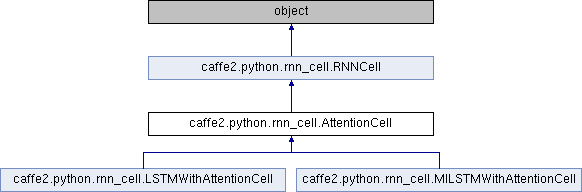Caffe2 - Python API A deep learning, cross platform ML framework
caffe2.python.rnn_cell.AttentionCell Class Reference
Inheritance diagram for caffe2.python.rnn_cell.AttentionCell:Public Member Functions

def __init__ (self, encoder_output_dim, encoder_outputs, encoder_lengths, decoder_cell, decoder_state_dim, attention_type, weighted_encoder_outputs, attention_memory_optimization, kwargs)

def get_attention_weights (self)

def prepare_input (self, model, input_blob)

def build_initial_coverage (self, model)

def get_state_names (self)

def get_output_dim (self)

def get_output_state_index (self)Public Member Functions inherited from caffe2.python.rnn_cell.RNNCell
def __init__ (self, name=None, forward_only=False, initializer=None)

def initializer (self)

def initializer (self, value)

def scope (self, name)

def apply_over_sequence (self, model, inputs, seq_lengths=None, initial_states=None, outputs_with_grads=None)

def apply (self, model, input_t, seq_lengths, states, timestep)

def apply_override (self, model, input_t, seq_lengths, timestep, extra_inputs=None)

def prepare_input (self, model, input_blob)

def get_output_state_index (self)

def get_state_names (self)

def get_state_names_override (self)

def get_output_dim (self)

Public Attributes

encoder_output_dim

encoder_outputs

encoder_lengths

decoder_cell

decoder_state_dim

weighted_encoder_outputs

encoder_outputs_transposed

attention_type

attention_memory_optimization

hidden_t_intermediate

coverage_weightsPublic Attributes inherited from caffe2.python.rnn_cell.RNNCell
name

recompute_blobs

forward_only

Detailed Description

Definition at line 1109 of file rnn_cell.py.

Member Function Documentation

 def caffe2.python.rnn_cell.AttentionCell.build_initial_coverage ( self, model )
```initial_coverage is always zeros of shape [encoder_length],
which shape must be determined programmatically dureing network
computation.

This method also sets self.coverage_weights, a separate transform
of encoder_outputs which is used to determine coverage contribution
tp attention.
```

Definition at line 1288 of file rnn_cell.py.

The documentation for this class was generated from the following file: